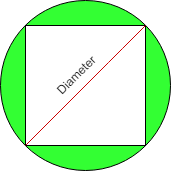# Find area of the Circle when the area of inscribed Square is given

• Difficulty Level : Hard
• Last Updated : 24 Nov, 2021

Given area of square inscribed in a circle as N, the task is to calculate the area of circle in which the square is inscribed.

Examples:

Input: N = 4
Output: 6.283

Input: N = 10
Output:  15.707

Approach:

Consider the below image:• Let the area of the square is ‘A’
• The side of the square is given by = A**(1/2)
• A right-angled triangle is formed by the two sides of the square and the diameter of the circle
• The hypotenuse of triangle will be diameter of circle
• The diameter of circle ‘D’ is calculated as ((A * A) + (A * A))**(1/2)
• The radius of circle ‘r’ is given by D/2
• The resultant area of the circle is pi*r*r

Below is the implementation of the above approach:

## C++

 `#include ` `#include` `#include ` `using` `namespace` `std;`   `// Function to calculate the area of circle` `double` `areaOfCircle(``double` `a)` `{` `    ``// declaring pi ` `    ``double` `pi=2*``acos``(0.0);` `      `  `    ``// Side of the square` `    ``double` `side = ``pow``(a,(1.0 / 2));`   `    ``// Diameter of circle` `    ``double` `D =``pow``( ((side * side) + (side * side)) ,(1.0 / 2));`   `    ``// Radius of circle` `    ``double` `R = D / 2;`   `    ``// Area of circle` `    ``double` `Area = pi * (R * R);`   `    ``return` `Area;`   `}` ` ``//Driver Code` `int` `main() {` `   `  `    ``double` `areaOfSquare = 4;` `    ``cout<

## Java

 `// Java code for the above approach` `import` `java.util.*;`   `class` `GFG` `{` `  `  `    ``// Function to calculate the area of circle` `    ``static` `double` `areaOfCircle(``double` `a)` `    ``{`   `        ``// Side of the square` `        ``double` `side = Math.pow(a, (``1.0` `/ ``2``));`   `        ``// Diameter of circle` `        ``double` `D = Math.pow(((side * side) + (side * side)),` `                            ``(``1.0` `/ ``2``));`   `        ``// Radius of circle` `        ``double` `R = D / ``2``;`   `        ``// Area of circle` `        ``double` `Area = Math.PI * (R * R);`   `        ``return` `Area;` `    ``}` `  `  `    ``// Driver Code` `    ``public` `static` `void` `main(String[] args)` `    ``{` `        ``double` `areaOfSquare = ``4``;` `        ``System.out.println(areaOfCircle(areaOfSquare));` `    ``}` `}`   `// This code is contribute by Potta Lokesh`

## Python3

 `# Python program for the above approach` `import` `math`   `# Function to calculate the area of circle` `def` `areaOfCircle(a):`   `    ``# Side of the square` `    ``side ``=` `a``*``*``(``1` `/` `2``)`   `    ``# Diameter of circle` `    ``D ``=` `((side ``*` `side) ``+` `(side ``*` `side))``*``*``(``1` `/` `2``)`   `    ``# Radius of circle` `    ``R ``=` `D ``/` `2`   `    ``# Area of circle` `    ``Area ``=` `math.pi ``*` `(R ``*` `R)`   `    ``return` `Area`   `# Driver Code` `areaOfSquare ``=` `4` `print``(areaOfCircle(areaOfSquare))`

## C#

 `// C# code for the above approach` `using` `System;`   `class` `GFG` `{` `  `  `    ``// Function to calculate the area of circle` `    ``static` `double` `areaOfCircle(``double` `a)` `    ``{`   `        ``// Side of the square` `        ``double` `side = Math.Pow(a, (1.0 / 2));`   `        ``// Diameter of circle` `        ``double` `D = Math.Pow(((side * side) + (side * side)),` `                            ``(1.0 / 2));`   `        ``// Radius of circle` `        ``double` `R = D / 2;`   `        ``// Area of circle` `        ``double` `Area = Math.PI * (R * R);`   `        ``return` `Area;` `    ``}` `  `  `    ``// Driver Code` `    ``public` `static` `void` `Main()` `    ``{` `        ``double` `areaOfSquare = 4;` `        ``Console.Write(areaOfCircle(areaOfSquare));` `    ``}` `}`   `// This code is contribute by Samim Hossain Mondal.`

## Javascript

 ``

Output

`6.283185307179588`

Time Complexity: O(1)
Auxiliary Space: O(1)

My Personal Notes arrow_drop_up
Recommended Articles
Page :